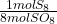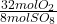## S8 +O2 = SO8 Given 80 mols of product, find the amount of each reactant in mols.

Question

S8 +O2 = SO8

Given 80 mols of product, find the amount of each reactant in mols.

in progress 0
5 months 2021-09-05T15:29:20+00:00 1 Answers 4 views 0

10 moles of S₈

320 moles O₂

Explanation:

• S₈ +O₂ → SO₈

The balanced equation is:

• S₈ + 32O₂ → 8SO₈

Now we can convert moles of SO₈ (the product) into moles of each reactant, using the stoichiometric coefficients of the balanced reaction:

• 80 mol SO₈ *= 10 mol S₈
• 80 mol SO₈ *= 320 mol O₂

In order for 80 moles of SO₈ to be produced, 10 moles of S₈ need to react with 320 moles of O₂.